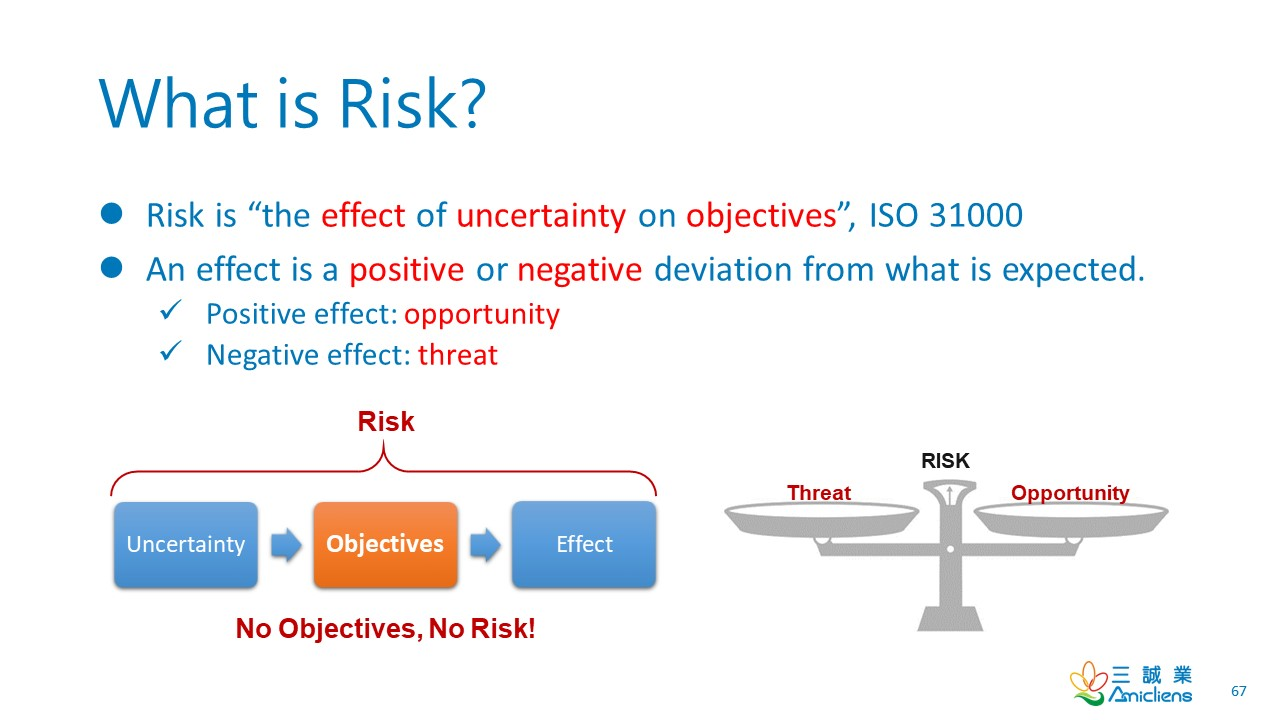# Risk = Threat x Vulnerability### Risk Exposure

Risk exposure is a measure of risk that is evaluated with consideration of all the risk factors. If the effect is evaluated with monetary value, risk exposure is an indicator of potential financial loss. A risk score is a common type of risk exposure.

Risk = Threat x Vulnerability

This formula is overly simplified and has been misunderstood for years. It is elaborated as follows:

• The Risk term in the formula should refer to “Risk Score” or “Risk Exposure.”
• The Threat term in the formula should refer to “The impact of a threat.”
• The Vulnerability term in the formula should refer to “The likelihood of the vulnerability being exploited.”
• The formula should be interpreted as “Risk Exposure is a function of the impact of a threat and the likelihood of the vulnerability being exploited.” As a result, the calculation doesn’t necessarily have to be multiplication.